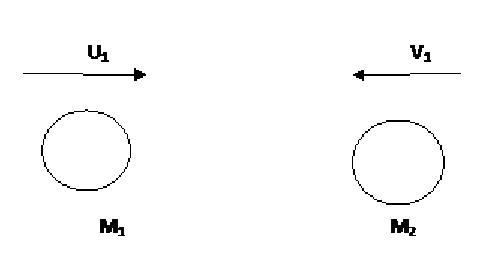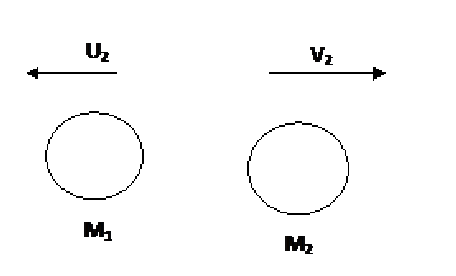Custom Search
If you want to discuss more on this or other issues related to Physics, feel free to leave a message on my Facebook page.

## Friday, February 26, 2010

### Conservation of kinetic energy in collisions

As we have seen in this post during a collision between two object the velocity of the two colliding objects changes. Hence you would agree that he kinetic energy of the objects would change.

We have also seen in this post that in all collisions the sum of linear momentum is a constant. This is the principle of conservation of linear momentum.  However as we are going to see in some collisions, elastic collisions, the sum of kinetic energy is also constant. That is the sum of the kinetic energies of the objects before the collision is the same as the sum of the kinetic energies after the collision.

We are now going to see an example of how to use this “principle” which i am going to call the “principle of conservation of kinetic energy”.

Fig 1 below shows two objects travelling towards each other and fig 2 shows the two objects separating after the two objects have separated.

So if the principle of conservation of kinetic energy applies then it means that Sum of kinetic energy before collision = sum of kinetic energy after collisionFig 1 Before collision

Before the collision

Sum of kinetic energy before collision = kinetic energy of object 1 + kinetic energy of object 2

= 1/2m1u12 + 1/2m2v12Fig 2 After collision

After the collision

Sum of kinetic energy after collision = kinetic energy of object 1 + kinetic energy of object 2

= 1/2m1u22 + 1/2m2v22

If you refer back to the principle then

Sum of kinetic energy before collision = sum of kinetic energy after collision

1/2m1u12 + 1/2m2v12   =   1/2m1u22 + 1/2m2v22

Example

Two balls of mass 1.0 kg are travelling towards each other at a speed of 5.0 m/s towards the right (ball 1)  and 5.0 m/s towards the left (ball 2) respectively. If after separation one of them moves moves off a speed of 5 m/s towards the right (ball 2 ) calculate the speed of the other ball.

Sum of kinetic energy before collision = sum of kinetic energy after collision

1/2m1u12 + 1/2m2v12   =   1/2m1u22 + 1/2m2v22

1/2*1.0*5.02 + 1/2*1.0*(-5.0)2   =   1/2*1.0*u22 + 1/2*1.0*5.02

Solving for u2 the velocity of object 1 after the collision

12.5+ 12.5 = 0.5*u22+12.5

12.5= 0.5*u22

12.5 /0.5 = 25 =u22

u22 = 25

There can be two solution to u2

First u2=-5 m/s and secondly u2= 5 m/s

Since the second ball is already travelling towards the right and according to the principle of conservation of linear momentum then the only solution is

u2=-5 m/s

1.I would like to know if kinetic energy was conserved during the collision , yes , it is conserved before and after but what about during that moment the collision takes place?

1.No. Kinetic Energy is doesn't remains conserved during the collision. During the collision, the total kinetic energy gets converted to potential energy.

2.I would like to ask why you are using v. The conservation law refers to the TOTAL energy. This is a scalar. The correct equation is 1/2 m s2, not 1/2 m v2. If you use a vector you are only looking at the KE in a particular direction and this will not be conserved. Momentum is the thing that is conserved as a vector. If a collision caused the balls to split at an angle to the trajectory, like it generally does, then the value for v will only give conservation of momentum and not energy.

3.You are right in thinking that the kinetic energy is a scalar quantity.
However velocity is a vector quantity. And the product of two vector quantities, velocity in this case, is a scalar quantity. For example work done( a scalar quantity) is the product of force and displacement (two vector quantities)

4.The product of two vectors is not always scalar. Cross product produces a vector and a dot product produces a scalar.